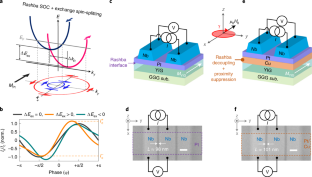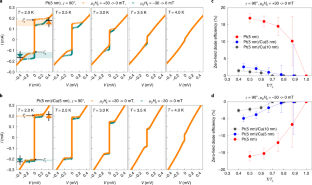Thank you for visiting nature.com. You are using a browser version with limited support for CSS. To obtain the best experience, we recommend you use a more up to date browser (or turn off compatibility mode in Internet Explorer). In the meantime, to ensure continued support, we are displaying the site without styles and JavaScript.

# Zero-field polarity-reversible Josephson supercurrent diodes enabled by a proximity-magnetized Pt barrier

### Subjects

An Author Correction to this article was published on 22 July 2022

## Abstract

Simultaneous breaking of inversion and time-reversal symmetries in a conductor yields a non-reciprocal electronic transport1,2,3, known as the diode or rectification effect, that is, low (ideally zero) conductance in one direction and high (ideally infinite) conductance in the other. So far, most of the diode effects observed in non-centrosymmetric polar/superconducting conductors4,5,6,7 and Josephson junctions8,9,10 require external magnetic fields to break the time-reversal symmetry. Here we report zero-field polarity-switchable Josephson supercurrent diodes, in which a proximity-magnetized Pt layer by ferrimagnetic insulating Y3Fe5O12 serves as the Rashba(-type) Josephson barrier. The zero-field diode efficiency of our proximity-engineered device reaches up to ±35% at 2 K, with a clear square-root dependence on temperature. Measuring in-plane field-strength/angle dependences and comparing with Cu-inserted control junctions, we demonstrate that exchange spin-splitting11,12,13 and Rashba(-type) spin-orbit coupling13,14,15 at the Pt/Y3Fe5O12 interface are key for the zero-field giant rectification efficiency. Our achievement advances the development of field-free absolute Josephson diodes.

This is a preview of subscription content, access via your institution

## Access options

\$32.00

All prices are NET prices.Fig. 1: Josephson φ0-junction with a proximity-magnetized Pt barrier.Fig. 2: Role of Rashba(-type) SOC in zero-field Josephson supercurrent rectification.Fig. 3: Magnetic field-strength and angle dependences of the Josephson supercurrent non-reciprocity.Fig. 4: Zero-field Josephson diode efficiency relying on the degree of magnetic proximity coupling.

## Data availability

Source data are provided with this paper. Other relevant data to this paper are available from the corresponding authors upon reasonable request.

## References

1. Onsager, L. Reciprocal relations in irreversible processes. I. Phys. Rev. 37, 405–426 (1931).

2. Kubo, R. Statistical-mechanical theory of irreversible processes. I. General theory and simple applications to magnetic and conduction problems. J. Phys. Soc. Jpn. 12, 570–586 (1957).

3. Rikken, G. L. J. A., Fölling, J. & Wyder, P. Electrical magnetochiral anisotropy. Phys. Rev. Lett. 87, 236602 (2001).

4. Tokura, Y. & Nagaosa, N. Nonreciprocal responses from non-centrosymmetric quantum materials. Nat. Commun. 9, 3740 (2018).

5. Wakatsuki, R. et al. Nonreciprocal charge transport in noncentrosymmetric superconductors. Sci. Adv. 3, e1602390 (2017).

6. Ando, F. et al. Observation of superconducting diode effect. Nature 584, 373–376 (2020).

7. Bauriedl, L. et al., Supercurrent diode effect and magnetochiral anisotropy in few-layer NbSe2 nanowires. Preprint at https://arxiv.org/abs/2110.15752 (2021).

8. Baumgartner, C. et al. Supercurrent rectification and magnetochiral effects in symmetric Josephson junctions. Nat. Nanotech. 17, 39–44 (2021).

9. Baumgartner, C. et al. Effect of Rashba and Dresselhaus spin-orbit coupling on supercurrent rectification and magnetochiral anisotropy of ballistic Josephson junctions. Preprint at https://arxiv.org/abs/2111.13983 (2021).

10. Pal, B. et al. Josephson diode effect from Cooper pair momentum in a topological semimetal. Preprint at https://arxiv.org/abs/2112.11285 (2021).

11. Buzdin, A. Direct coupling between magnetism and superconducting current in the Josephson φ0 junction. Phys. Rev. Lett. 101, 107005 (2008).

12. Bergeret, F. S. & Tokatly, I. V. Theory of diffusive φ0 Josephson junctions in the presence of spin–orbit coupling. Europhys. Lett. 110, 57005 (2015).

13. Konschelle, F., Tokatly, I. V. & Bergeret, F. S. Theory of the spin-galvanic effect and the anomalous phase shift φ0 in superconductors and Josephson junctions with intrinsic spin-orbit coupling. Phys. Rev. B 92, 125443 (2015).

14. Bezuglyi, E. V., Rozhavsky, A. S., Vagner, I. D. & Wyder, P. Combined effect of Zeeman splitting and spin-orbit interaction on the Josephson current in a superconductor-two-dimensional electron gas-superconductor structure. Phys. Rev. B 66, 052508 (2002).

15. Reynoso, A. A., Usaj, G., Balseiro, C. A., Feinberg, D. & Avignon, M. Anomalous Josephson current in junctions with spin polarizing quantum point contacts. Phys. Rev. Lett. 101, 107001 (2008).

16. Neamen, D. A. Semiconductor Physics and Devices: Basic Principles 4th edn (McGraw-Hill, 2012).

17. Muller, R. S., Kamins, T. I. & Chan, M. Device Electronics for Integrated Circuits 3rd edn (John Wiley & Sons, Inc., 2003).

18. Rashba, E. I. Svoistva poluprovodnikov s petlei ekstremumov. I. Tsiklotronnyi i kombinirovannyi rezonans v magnitnom pole, perpendikulyarnom ploskosti petli. [Properties of semiconductors with an extremum loop. 1. Cyclotron and combinational resonance in a magnetic field perpendicular to the plane of the loop] Fiz. Tverd. Tela (Leningr.) 2, 1224–1238 (1960).

19. Manchon, A., Koo, H. C., Nitta, J., Frolov, S. M. & Duine, R. A. New perspectives for Rashba spin-orbit coupling. Nat. Mater. 14, 871–882 (2015).

20. Szombati, D. B. et al. Josephson φ0-junction in nanowire quantum dots. Nat. Phys. 12, 568–572 (2016).

21. Assouline, A. et al. Spin-orbit induced phase-shift in Bi2Se3 Josephson junctions. Nat. Commun. 10, 126 (2019).

22. Mayer, W. et al. Gate controlled anomalous phase shift in Al/InAs Josephson junctions. Nat. Commun. 11, 212 (2020).

23. Strambini, E. et al. A Josephson phase battery. Nat. Nanotechnol. 15, 656–660 (2020).

24. Golubov, A. A., Kupriyanov, M. Y. & Il’ichev, E. The current phase relation in Josephson junctions. Rev. Mod. Phys. 76, 411–469 (2004).

25. Vlietstra, N. et al. Exchange magnetic field torques in YIG/Pt bilayers observed by the spin-Hall magnetoresistance. Appl. Phys. Lett. 103, 032401 (2013).

26. Žutić, I., Matos-Abiague, A., Scharf, B., Hanan Dery, H. & Belashchenko, K. Proximitized materials. Mater. Today 22, 85–107 (2019).

27. Tanaka, T. et al. Intrinsic spin Hall effect and orbital Hall effect in 4d and 5d transition metals. Phys. Rev. B 77, 165117 (2008).

28. Diez-Merida, J. et al. Magnetic Josephson junctions and superconducting diodes in magic angle twisted bilayer graphene. Preprint at https://arxiv.org/abs/2110.01067 (2021).

29. Jeon, K.-R. et al. Giant transition-state quasiparticle spin-Hall effect in an exchange-spin-split superconductor detected by nonlocal magnon spin transport. ACS Nano 14, 15874–15883 (2020).

30. Zhang, X.-P., Bergeret, F. S. & Golovach, V. N. Theory of spin Hall magnetoresistance from a microscopic perspective. Nano Lett. 19, 6330–6337 (2019).

31. Ilić, S. & Bergeret, F. S. Theory of the supercurrent diode effect in Rashba superconductors with arbitrary disorder. Phys. Rev. Lett. 128, 177001 (2022).

32. Wu, H. et al. The field-free Josephson diode in a van der Waals heterostructure. Nature 604, 653–656 (2022).

33. Buzdin, A. I. Proximity effects in superconductor-ferromagnet heterostructures. Rev. Mod. Phys. 77, 935–976 (2005).

34. Bergeret, F. S. & Tokatly, I. V. Singlet-triplet conversion and the long-range proximity effect in superconductor-ferromagnet structures with generic spin dependent fields. Phys. Rev. Lett. 110, 117003 (2013).

35. Barone, A. & Paterno, G. Physics and applications of the Josephson effect 2nd edn (John Wiley & Sons, 1982).

## Acknowledgements

This work was supported by the Alexander von Humboldt Foundation.

## Author information

Authors

### Contributions

K.-R.J. and S.S.P.P conceived and designed the experiments with the help of T.K. K.-R.J. fabricated the lateral Josephson junctions and Hall-bar devices with help from J.-K.K., J.Y. and J.-C.J., and carried out the transport measurements with help of J.-C.J. H.H. contributed to the structural analysis. K.-R.J. performed the data analysis with the guidance of A.C. and T.K. K.-R.J. and S.S.P.P. wrote the manuscript with input from all the other co-authors.

### Corresponding authors

Correspondence to Kun-Rok Jeon or Stuart S. P. Parkin.

## Ethics declarations

### Competing interests

The authors declare no competing interests.

## Peer review

### Peer review information

Nature Materials thanks Jacobo Santamaria and the other, anonymous, reviewer(s) for their contribution to the peer review of this work.

Publisher’s note Springer Nature remains neutral with regard to jurisdictional claims in published maps and institutional affiliations.

## Extended data

### Extended Data Fig. 1 X-ray structural characterisation of Pt/YIG/GGG(111).

a, θ-2θ X-ray diffraction (XRD) scans of Pt/YIG/GGG(111) samples with different Pt thicknesses tPt = 3, 5 and 10 nm. The Pt(111) thin films are crystallized on YIG/GGG(111) substrates. b, Detailed θ-2θ XRD scan near the GGG(444) peak, from which one can determine the epitaxy of the YIG(111) film on the GGG(111) wafer. c, X-ray reflection (XRR) profiling of the Pt/YIG(111)/GGG(111) samples with different tPt. Note that the respective tPt was estimated from the oscillations of each of the XRR curves.

### Extended Data Fig. 2 Estimation of exchange spin-splitting from AHE measurements.

a, Schematic illustration of Pt Hall-bar device and measurement geometry. If the Pt metal layer is proximity-magnetised by the ferrimagnetic insulating YIG underneath it, non-zero anomalous Hall resistivity ρAH detectable under OOP magnetic field μ0H provides a straightforward measurement of how large the exchange spin-splitting is induced in the Pt layer. Here ρAH = RAHtPt, where RAH is the anomalous Hall resistance and tPt is the Pt thickness. b, Temperature T evolution of the longitudinal Pt resistivity ρPt for tPt = 3, 5 and 10 nm. c, ρAH versus μ0H plots for the tPt = 3, 5 and 10 nm devices, taken at T = 2 K. The inset shows the measured ρAH(tPt) data and a fit to estimate the imaginary part of the spin-mixing conductance Gi, which is directly proportional to the exchange spin-splitting in the proximity-magnetised Pt. d, Typical magnetic hysteresis curves of mYIG(μ0H) and mYIG(μ0H) (inset) for the bare YIG(200 nm) at 2 K.

### Extended Data Fig. 3 Absence of Josephson supercurrent rectification in the Cu-only JJ.

a, Schematic of the junction geometry and measurement configuration of the Nb/Cu/Nb reference Josephson junction (JJ). b, Zero-field current-voltage I-V curves of the 5-nm-thick Cu JJ for two different YIG magnetization MYIG (// ±x-axis) below the junction’s superconducting transition temperature Tc. Note that μ0H =± 30 mT, much larger than the coercive field of YIG29, is first applied along the x-axis and then returned to zero to initialise the remanent-state MYIG in the ±x-direction. c, Summary of the zero-field diode efficiency $$Q_{\mu _0H = 0} = \left[ {\frac{{I_c^ + - \left| {I_c^ - } \right|}}{{I_c^ + + \left| {I_c^ - } \right|}}} \right]_{\mu _0H = 0} = \left[ {\frac{{{\Delta}I_c}}{{2I_c^{avg}}}} \right]_{\mu _0H = 0}$$ as a function of normalized temperature T/Tc for the Cu-only reference JJ.

### Extended Data Fig. 4 Asymmetric OOP magnetic-field interface patterns reflecting the existence of non-zero φ0.

a, Out-of-plane (OOP) magnetic-field interference patterns Ic(μ0H) at zero in-plane (IP) field μ0H = 0 for the 3-nm-thick Pt Josephson junction (JJ). Note that μ0H =± 30 mT, much larger than the coercive field of YIG29, is first applied along the x-axis and then returned to zero to initialize the remanent-state MPt in the ±x-direction. The insets show current–voltage I–V curves of the 3-nm-thick Pt JJ at μ0H= ± 10 mT. In the left (right) inset, the remanent-state MPt is in the positive (negative) x-direction. Note that the strong suppression of Ic under μ0H is due to the large effective junction area of magnetic flux penetration35. b, Data equivalent to a but for the Cu-only JJ, where Ic(μ0H) is almost independent of the remanent-state MPt. c, Simulated asymmetric OOP magnetic-field interference patterns $$I_c\left( {\frac{{{\Phi}_{\mu _0H_ \bot }}}{{{\Phi}_0}}} \right)$$ for a diffusive JJ. Here phase variations are induced along the x-direction because of changes in the Rashba SOC due to finite structural disorder21. In this simulation, we assume $$\frac{{I_{c1}}}{{I_{c2}}}$$ = 5 and a sinusoidal spatial variation of the Rashba SOC as αR(x) = α0A(x) = $$\alpha _0\left( {1 - 0.95\sin \left[ {\frac{x}{w}} \right]} \right)$$ for Φμ0H = 0, where α0 = 0.002 eVÅ. d, Data equivalent to c, but for the case of weak SOC α0 = 0.0002 eVÅ.

## Source data

### Source Data Fig. 2

Statistical source data.

### Source Data Fig. 3

Statistical source data.

### Source Data Fig. 4

Statistical source data.

### Source Data Extended Data Fig. 1

Statistical source data.

### Source Data Extended Data Fig. 2

Statistical source data.

### Source Data Extended Data Fig. 3

Statistical source data.

### Source Data Extended Data Fig. 4

Statistical source data.

## Rights and permissions

Reprints and Permissions

• ### Superconducting diodes with no magnetic field

• Jacobo Santamaria

Nature Materials (2022)

## Search